[BACK]Computer Modeling in Engineering & SciencesDOI: 10.32604/cmes.2021.016133

ARTICLE

Fatigue Topology Optimization Design Based on Distortion Energy Theory and Independent Continuous Mapping Method

Faculty of Materials and Manufacturing, Beijing University of Technology, Beijing, 100124, China
*Corresponding Author: Hongling Ye. Email: yehongl@bjut.edu.cn
Received: 09 February 2021; Accepted: 16 April 2021

Abstract: Fatigue failure is a common failure mode under the action of cyclic loads in engineering applications, which often occurs with no obvious signal. The maximum structural stress is far below the allowable stress when the structures are damaged. Aiming at the lightweight structure, fatigue topology optimization design is investigated to avoid the occurrence of fatigue failure in the structural conceptual design beforehand. Firstly, the fatigue life is expressed by topology variables and the fatigue life filter function. The continuum fatigue optimization model is established with the independent continuous mapping (ICM) method. Secondly, fatigue life constraints are transformed to distortion energy constraints explicitly by taking advantage of the distortion energy theory. Thirdly, the optimization formulation is solved by the dual sequence quadratic programming (DSQP). And the design scheme of lightweight structure considering the fatigue characteristics is obtained. Finally, numerical examples illustrate the practicality and effectiveness of the fatigue optimization method. This method further expands the theoretical application of the ICM method and provides a novel approach for the fatigue optimization problem.

Keywords: Fatigue optimization; distortion energy theory; S-N curve; independent continuous mapping method

1  Introduction

Continuum structural topology optimization is the most difficult optimization problem in structural topology optimization. Topology optimization has four important significances including searching for the best transmission path of forces, forming the optimal distribution of structural material, optimizing performance of the structure, and achieving structural lightweight. Many researchers devote themselves to topology optimization because of novel design , or lightweight structure in the engineering application [4,5]. The typical continuum topology optimization methods include variable density method , homogenization method , evolutionary structural optimization method , level set method [9,10], variable thickness method , moving morphable components (MMC) method [12,13], moving morphable void (MMV) method , phase field method [15,16], and the independent continuous mapping (ICM) method  etc.

Structural fatigue may occur when engineering structures are subjected to periodic or random loads. In this case, the structural stress is less than the material strength limit. Due to the absence of obvious signal and the uncertainty of the occurrence, the fatigue failure is a great safety hazard. To improve the anti-fatigue performance of the structure, it is important to design the engineering components with fatigue topology optimization. There have been some researches on fatigue topology optimization but in a few quantity. In the early researches, the crack initiation was used to indicate that the structural fatigue failure had occurred, and the fatigue life constraints were normally transformed to the stress constraints since it was convenient to calculate. For high cycle fatigue optimization, Mrzyglod et al.  built a lightweight topology optimization model with fatigue life constraints. Fatigue life constraints were transferred to stress constraints with the Dang Von criterion. The equivalent stress was used to express fatigue failure based on ANSYS. In low cycle fatigue topology optimization, Desmorat et al.  optimized a low cycle fatigue model with minimum volume as an objective. Relative energy density function and Lemaitre’s law were used to transform fatigue life constraints to stress constraints. The fatigue failure was calculated by stress constraints with the cyclic elasto-plasticity law.

With the progress of the manufacturing industry, the service life of industrial products is increasing. More topology optimization problems are considered with high cycle fatigue life constraints [20,21]. For the expression of structural failure, most researchers choose the accumulated damage criterion to describe the fatigue life constraints directly. Holmberg et al.  and Oest et al.  built the lightweight fatigue topology optimization model. They chose the same criterion, Palmgren-Miner’s rule, to calculate the structural accumulated damage while the stress calculation methods were different. The former chose a fictitious load spectrum, the latter referred to a linear log-log S-N curve. Lee et al.  transformed the load spectrum in frequency domain to the time domain. And a lightweight fatigue topology optimization model was established. The structural accumulated damage was expressed by equivalent stress with the S-N curve. The long-time span fatigue loads were simplified into equivalent stress by calculating power density function with Dirlik method, narrow solution, etc., Chen et al.  developed a density filter SIMP method to optimize the component with the fatigue loading. The singularity issue is circumvented by the penalized stress. And the lightweight design is obtained with the constraint of the maximum fatigue damage.

The fatigue failure is a local phenomenon, which means the fatigue life of each unit should satisfy the fatigue life constraints. Some efficient methods were introduced to reduce computation, like the P-norm function , the q-p relaxed approach , and K-S function , etc.

The ICM method is effective to solve the structural topology optimization problems, especially in static, frequency and buckling problems [17,29,30]. However, a few studies focus on fatigue topology optimization problems. Therefore, inspired by ICM method, the lightweight continuum topology optimization model with the fatigue life constraints is established in this paper. The distortion energy theory and the S-N curve are utilized to transform fatigue life constraints are into distortion energy constraints explicitly. And the DSQP method is utilized to reduce computation and solve the topology optimization model.

This paper consists of seven sections. In Section 2, three features in ICM method are demonstrated. In Section 3, the fatigue life filter function is introduced to establish fatigue topology optimization model, and the fatigue life constraints are explicitly transformed to distortion energy constraints. In Section 4, the process of solving strategies with the topology optimization model is represented. In Section 5, the program flow of the optimization algorithm is presented. In Section 6, three numerical examples are presented to demonstrate the validity of the fatigue optimization method. Finally, the conclusions are obtained.

2  ICM Method

ICM method, which is a continuous topology optimization design method proposed by Professor Sui in 1996 , with three salient features consist of lightweight design, high computational efficiency, and the filter function.

Lightweight design is the consistent optimization goal of ICM method because of the high economic value in the aerospace industry, automobile industry, etc. This is of great significance for saving production cost, reducing use cost, improving product mechanical performance, etc.

The high computational efficiency is realized by two transformations of optimization model. First, the discrete optimization model is transformed into a continuous optimization model by introducing the independent continuous variable. In ICM method, the discrete variables that are 0/1 are converted to continuous variables that belong to [0,1] and inverse them back to discrete variables after optimization. Second continuous optimization model is transformed into the quadratic programming optimization model by introducing the DSQP method. The DSQP method is the combination of duality theory and the SQP algorithm. This method could transform the constraints into the objective and form a dual optimal model. Therefore, the amount of constraints is reduced, which leads to a reduction in computation.

The filter functions establish the relationship between topology variables and physic properties or geometric dimensions. The expressions of filter function will determine the establishment and solution of topology optimization. Further, it will have an impact on the performance of the optimized structure. It is the key point to establish the relationship between discrete variables and continuous variables. In mathematics concept, the filter function is the result of the continuous infinite approximation of x=1,0y1 and y=0,0x1, and it is a monotone increasing function with differentiability in the interval (0,1].

The differences among the filter functions directly affect the computational efficiency and the optimization results. We usually use power functions, f(ti)=tiα, α1, as the form of filter functions. The i presents the current unit number and i=1,,N, N is the number of units. In Fig. 1, the v presents a kind of unit performance, the v0 presents the limitation of v.Figure 1: Filter function

Mass filter function fW(ti), fatigue life filter function fL(ti), stress filter function fσ(ti), and the dynamic stiffness matrix filter function fkL(ti) are introduced to identify the relationship between unit parameters and design variables.

σ¯=fσ(ti)σ¯0,L_=L_0fL(ti),wi=fW(ti)wi0 (1)

σ¯, the “extend allowable stress” function, is expressed by the stress filter function, which is transformed from the 0/1 discrete state to the continuous state in the interval (0,1]. σ¯0 is the allowable stress. fσ(ti) = tiασ is the stress filter function, ασ = 3. L_0 is the allowable fatigue life. And L_ is the extended allowable fatigue life, which is expressed in the same way with σ¯. fL(ti) = tiαL is the fatigue life filter function, αL = 1. wi is the unit mass, wi0 is the inherent unit mass, fW(ti) = tiαw is the mass filter function, αw = 3.

3  Mathematical Model

3.1 Fatigue Life Filter Function

Fatigue failure exists widely in actual projects. When fatigue failure occurs, the structural component usually fails before alternating stress reaches the allowable value of structural stress. There is no obvious warning when an accident occurs. And it is difficult to prevent in advance, resulting in great losses. To improve the structural fatigue performance. The fatigue failure is considered in structural concept design.

The lightweight fatigue topology optimization model is presented.

{FindtENmakeW=i=1Nwimins.t.LiL_i(0<ti_ti1;i=1,,N) (2)

t is the vector of topology variables, and t={t1,t2,,tN}. W is the structural mass. Li is the unit fatigue life. L_i is the unit extended allowable fatigue life. Structure failure occurs when LiL_i, the structure remains stable when LiL_i.

In fatigue topology optimization, the unit fatigue life of the structure should be greater than the unit extended allowable fatigue life:

LiL_i (3)

And Eq. (1) has been substituted into Eq. (3), Eq. (3) can be written as follows:

LiL_i0fL(ti) (4)

Above all, the unit extends allowable fatigue life is identified by the filter function of the unit allowable fatigue life in the fatigue topology optimization. Then we get the lightweight fatigue topology optimization model as follows:

{FindtENmakeW=i=1Nfw(ti)wi0mins.t.LiL_i0fL(ti)(0<ti_ti1;i=1,,N) (5)

3.2 Transformation of Fatigue Life Constraints

To establish the sign of fatigue failure, the structural damage accumulation theory is introduced to represent the structural damage. The fatigue damage can be linearly accumulated with the Miner rule and defined as follows:

D=Dj = j=1kljLj (6)

Lj represents the fatigue life of the j-th stress level, lj represents the actual cycle number for the j-th stress level. Dj is the structural damage of the j-th stress level. When D is equal to 1, the structure is damaged.

With the Miner rule, the sign of fatigue failure is obtained. The mathematical expression of structural failure is established. But in the physical phenomenon, the fact of fatigue failure is the release of structure energy. It is important to obtain the physical expression of structural failure. The relationship between fatigue life and distortion energy is presented by the S-N curve and distortion energy theory. First, the fatigue life is transformed to the structural peak stress with S-N curve. Then the structural peak stress is transformed to distortion energy by distortion energy theory.

3.2.1 Relationship between Fatigue Life and Structural Peak Stress

The fatigue life is transferred to structural peak stress with S-N curve and structural damage accumulation theory. The form of the S-N curve is formulated by the power function and defined.

σLβL=C (7)

β and C are material constants, σL is the structural peak stress subject to cyclic load. L is the fatigue life.

The S-N curve is used to make the fatigue topology optimization constraint explicitly. And the optimized model in Eq. (5) is transformed as:

{FindtENmakeW=i=1Nfw(ti)wi0mins.t.σiLβ(Li)fL(ti)σiLβ(L_i0)(0<ti_ti1;i=1,,N) (8)

σiL(Li) is the unit structural cyclic peak stress corresponding to fatigue life. σiL(L_i0) is the unit structural cyclic peak stress corresponding to allowable fatigue life.

3.2.2 Relationship between Structural Peak Stress and Distortion Energy

The distortion energy theory is presented as follows:

σi=[(σi1σi2)2+(σi2σi3)2+(σi3σi1)2]/2,ei = (1+μ)σi2Vi3E (9)

σi is the unit maximum element stress, σi1,σi2,σi3 are the unit principal stress. Vi is the unit structure volume. ei is the unit structural strain energy. In distortion energy theory, the distortion energy is the main state variable of material yield, Eq. (9) can be written as follows:

eif(1+μ)σ¯i2Vi3E (10)

In this inequation, eif presents the unit distortion energy, μ presents Poisson’s ratio, E presents modulus of elasticity, σ¯i presents unit extend allowable stress.

The unit distortion energy eif is a part of the unit structural strain energy ei, so Eq. (11) is obtained.

eifei (11)

In order to keep the results safe, the unit structural strain energy is the substitute for the unit distortion energy.

ei(1+μ)σ¯i2Vi3E (12)

The cyclic load in fatigue topology optimization is dynamic. The unit structural strain energy ei is replaced by the unit dynamic strain energy eLi. The coefficient ζ is introduced to express the relationship between dynamic strain energy and structural strain energy. The dynamic strain energy is expressed as:

eLi=ξei,eLie¯Li (13)

e¯Li is the unit allowable dynamic strain energy corresponding to the structural peak stress.

Based on the relation between the unit structural strain energy ei and the unit dynamic strain energy eLi, the expression of distortion energy constraints is obtained.

eLiξ(1+μ)σ¯i2Vi3E (14)

Then the dynamic stiffness matrix filter function is introduced as follows:

kLi=fkL(ti)kLi0 (15)

kLi is the unit dynamic stiffness matrix, kLi0 is the unit inherent dynamic stiffness matrix. fkL(ti) = tiαkξ is the fatigue life filter function, αk = 3. With ui=ki1Fi, where ui and Fi represent the unit displacement vector and the unit nodal force vector respectively, we can get:

eLi=ξei=ξ12uiTkiui=ξFiT(ki)1Fi2=FiTFi2(kiξ)1 (16)

ki is the unit stiffness matrix. We define kLi=kiξ, and the Eq. (16) can be written as follows:

eLi=FiT(kLi)1Fi2 (17)

tv are the topology variables for the v-th iteration to be, therefore, Eq. (18) is obtained from Eq. (15).

kLiv=fkL(ti(v))kLi0 (18)

Eq. (15) is substituted into Eq. (18), we have

kLi=fkL(ti)fkL(ti(v))kLiv (19)

And then, we can get:

eLi=fkL(ti(v))FiT(kLi)1Fi2fkL(ti) (20)

The hypothesis of static determination is introduced. The internal forces Fi remain unchanged, which means Fi = Fi(v). Then we get the implicit expression of dynamic strain energy.

eLi=fkL(ti(v))fkL(ti)eLi(v) (21)

To obtain the explicit expression of the unit dynamic strain energy, the stress constraints in Eq. (8) are deformed.

When fatigue failure occurs, the unit structural peak stress is smaller than the unit allowable structure stress, that is:

σiL(L_i0)<σ¯i (22)

Based on the distortion energy theory, we transform Eq. (22), and get:

(1+μ)σiL2(L_i0)Vi3E<(1+μ)σ¯i2Vi3E (23)

The constraint in Eq. (8) can be written as:

(1+μ)σiL2(Li)Vi3E<fL2β1(ti)(1+μ)σiL2(L_i0)Vi3E (24)

The unit structure distortion energy corresponding to σiL(L_i0) is smaller than the unit allowable dynamic strain energy. In this condition, the unit allowable dynamic strain energy is used to replace the unit distortion energy.

(1+μ)σiL2(L_i0)Vi3E<fL2β1(ti)e¯Li (25)

Both sides of Eq. (25) are summed with the number of units i, respectively.

i=1neLii=1ne¯LifL2β1(ti) (26)

Eq. (21) is substituted into Eq. (26), and we can get:

i=1nfkL(ti(v))teLi(v)fkL(ti)fL2β1(ti)e¯Li (27)

The filter functions fw(ti)=tiαw, fk(ti)=tiαk, and fkL(ti)=tiαkξ, fL(ti)=tiαL are introduced, and Eq. (27) can be written as:

i=1nξfkL(ti(v))eLi(v)ti2αLβ1+αke¯Li (28)

For the convenience of proof, we set:

xi=1ti2αLβ1+αk (29)

The constraints can be explicitly expressed as Eq. (30):

i=1nBixie¯Li (30)

where Bi=ξfk(ti(v))eLi(v). At this time, all the fatigue life constraints have been transformed into dynamic strain energy constraints.

4  Solution

4.1 Standardization of the Objective

The second-order Taylor expansion is introduced to standardize the objective. According to the topology optimization formulation, the objective is shown as Eq. (31):

W=i=1Nfw(ti)wi0min (31)

Eq. (29) is substituted into Eq. (32), we can obtain:

fw(ti)=tiαk=xiαk2αLβ1+αk (32)

Then we set A=αk2αLβ1+αk, the objective function can be expressed as:

W=i=1Nfw(ti)wi0=xiAwi0 (33)

The objective is expanded by quadratic Taylor:

Wxi=AxiA1 (34)

2Wxi2=A(A1)xiA2 (35)

W(x)=W(x0)+i=1NWxi|xi0(xixi0)+12i=1N2Wxi2|xi0(xixi0)2=i=1N(x0)Awi0+i=1NA(xi0)A1xiwi0i=1NA(xi0)Awi0+12i=1NA(A1)(xi0)Awi0+12i=1NA(A1)(xi0)A2xi2wi012i=1NA(A1)(xi0)A1xiwi0 (36)

The constant terms can be ignored, so the W can be written as follows:

W=i=1NA(xi0)A1xi+12i=1NA(A1)(xi0)A2xi212i=1NA(A1)(xi0)A1xi=i=1NA(A+1)(xi0)A1wi0xi+12i=1NA(A1)(xi0)A2wi0xi2 (37)

Therefore, a standard sequential quadratic programming model can be obtained:

{Findx=(x1,,xN)TmakeW=bixi2+aiximins.t.i=1nBixie¯Li(1xix¯i;i=1,,N) (38)

where ai=i=1NA(A+1)(xi0)A1wi0, bi=12i=1NA(A1)(xi0)A2wi0, A=αk2αLβ1+αk, Bi=ξfk(ti(v))eLi(v).

4.2 Solution of the Optimization Model

The number of design variables is larger than constraints in fatigue topology optimization. According to dual theory, the above topology optimization formulation programming is transformed into dual programming, as shown in Eq. (39):

{FindλEmakeΦ(λ)maxs.t.λi0(i=1,..,N) (39)

where L(x,λ)=i=1N(bixi2+aixi)+λ(i=1NBixie¯), Φ(λ)=min1xixi¯(L(x,λ)), λ is Lagrange multiplier.

In Eq. (39), the objective is approximated by the second-order Taylor expansion, and the dual theory is utilized to solve the sensitivity of the objective.

Then Φ(λ) is written as:

Φ(λ)=12λTDλ+HTλ (40)

where HT=[Φ(λ0)Tλ0T2Φ(λ0)], D=2Φ(λ0). λ, which is explicitly expressed in H, is eliminated:

2Φ(λ)λλkλk=i=1NBiBik2biλk=i=1NBi2biBikλk=iIaBi2bi(2bixi+ai) (41)

Φ(λ)λ2Φ(λ)λλkλk=i=1NBixie¯iIaBi2bi(2bixi+ai) (42)

So, we can get Eq. (43):

H=i=1NBixi+e¯+iIaBi2bi(2bixi+ai),Dk=iIaBiBik2bi (43)

The quadratic programming model is obtained after Φ(λ) quadratic approximation and the constant term can be ignored.

{FindλEmakeΦ(λ)=12λTDλ+HTλmins.t.λi0(i=1,,N) (44)

After the quadratic programming has been solved, then the Eq. (44) is updated, and the next iteration is carried out until:

||x(v+1)x(v)||||x(v)||ε (45)

Then the iteration can be terminated. The x* is the optimized solution of Eq. (39). Then t* can be calculated from Eq. (29). We can get the optimized structure until:

ΔW=|(W(v+1)W(v))W(v+1)|ε (46)

W(v), W(v+1) are the previous iteration structure mass and the current iteration structure mass, respectively. ε is convergence precision, ε = 0.001.

5  Program Flow of Optimization Algorithm

The fatigue optimization method is applied to MSC.Patran software platform with MSC.Natran and MSC.Fatigue solver. PCL language is used to realize the continuum fatigue topology optimization. The details of the fatigue topology optimization procedure are given, and the algorithm flowchart is shown in Fig. 2.Figure 2: Program flow of fatigue topology optimization algorithm

Step 1: Establish the continuum fatigue topology optimization model based on MSC.Patran.

Step 2: Set an optimized objective, fatigue constraints. Initialize the element topological values.

Step 3: Carry out the fatigue analysis with MSC.Patran.

Step 4: Form the topology optimization with dynamic strain energy constraints.

Step 5: Solve the topology optimization with the dual sequence quadratic programming (DSQP) method. Get the continuous topology optimization structure.

Step 6: Judge convergence of the optimized structural mass. If the results satisfy Eq. (46). Then the topology optimization continues. Otherwise, obtain the new topology values and update the FEM model, back to Step 3.

Step 7: Obtain the discrete topological variables with the inverse threshold. Form the discrete topological structure.

Step 8: Carry out the fatigue analysis with MSC.Patran.

Step 9: Judge fatigue life of the optimized results. If it satisfies the fatigue life constraints, obtain the optimized structure, and end the calculation. Otherwise, modify the inverse threshold and go to Step 7.

6  Numerical Examples

Three examples are presented to test the fatigue optimization method. The form of cyclic load in numerical examples is the sine function, which is shown in Fig. 3. Young’s modulus E=210GPa, Poisson’s ratio μ=0.25.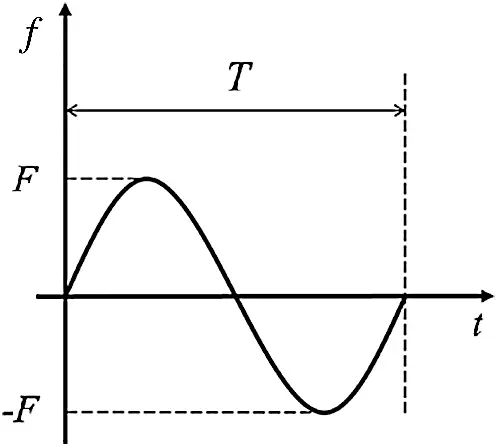Figure 3: Form of concentrated dynamic load

6.1 Example 1

The design domain is a cantilever with the size of 10 mm×4 mm×0.4 mm, which is shown in Fig. 4A. A cyclic load is applied to the middle of the right side. The force is applied to three nodes to avoid the stress concentration. The peak value of cyclic load is F = 420 N, ρ = 1 kg/cm3. The original structure mass is 16 g. Fatigue life constraint is 500 cycles.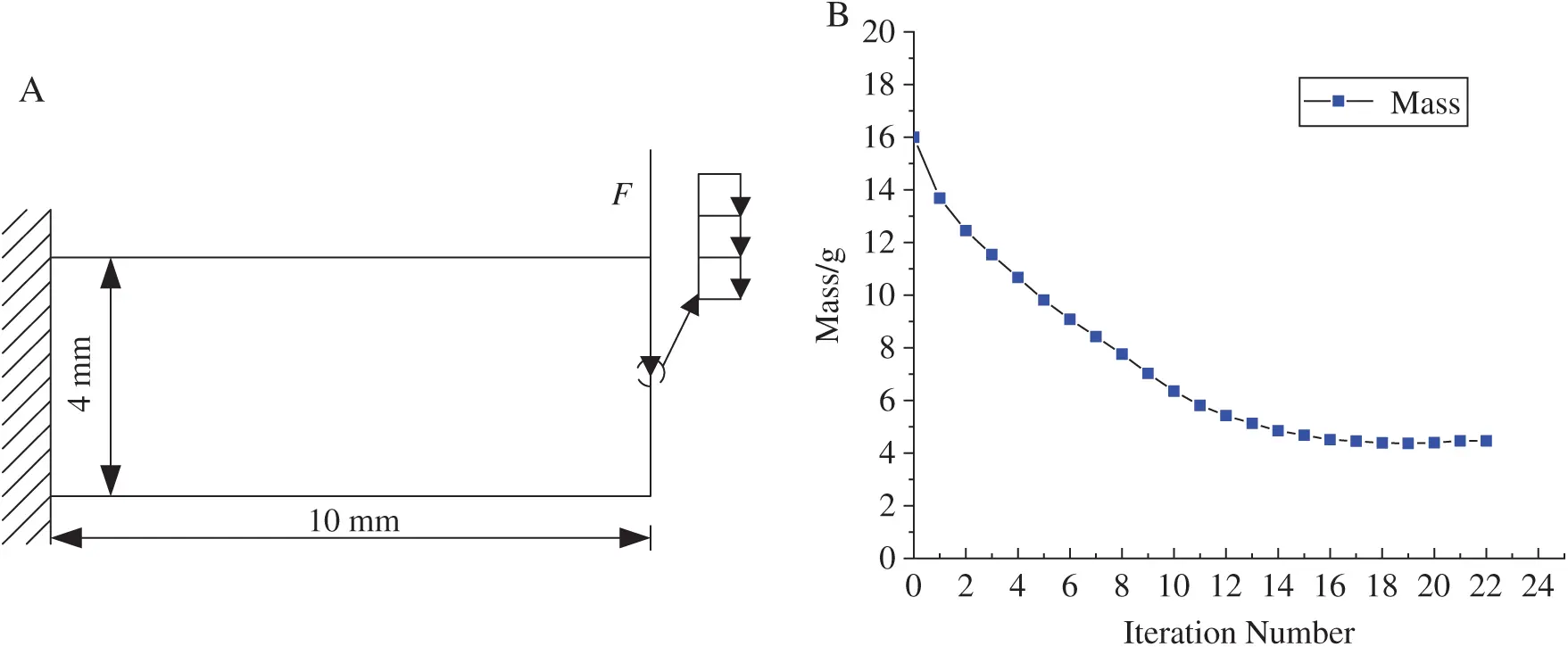Figure 4: (A) Basic structure. (B) Iteration history curve of optimized structure

The iterative history of the mass is shown in Fig. 4B. The structure mass decreases gradually until the convergence precision is satisfied. The structure mass decreases from 16 g to 4.47 g.

Tab. 1 gives the configurations and stress nephograms of topology optimization structure Comparing the basic structure and optimized structure. Although there is a certain increase in the maximum structural Von mises stress, we think the optimized structure is reasonable due to the fatigue life of optimized structure is 582 that satisfies the constraint.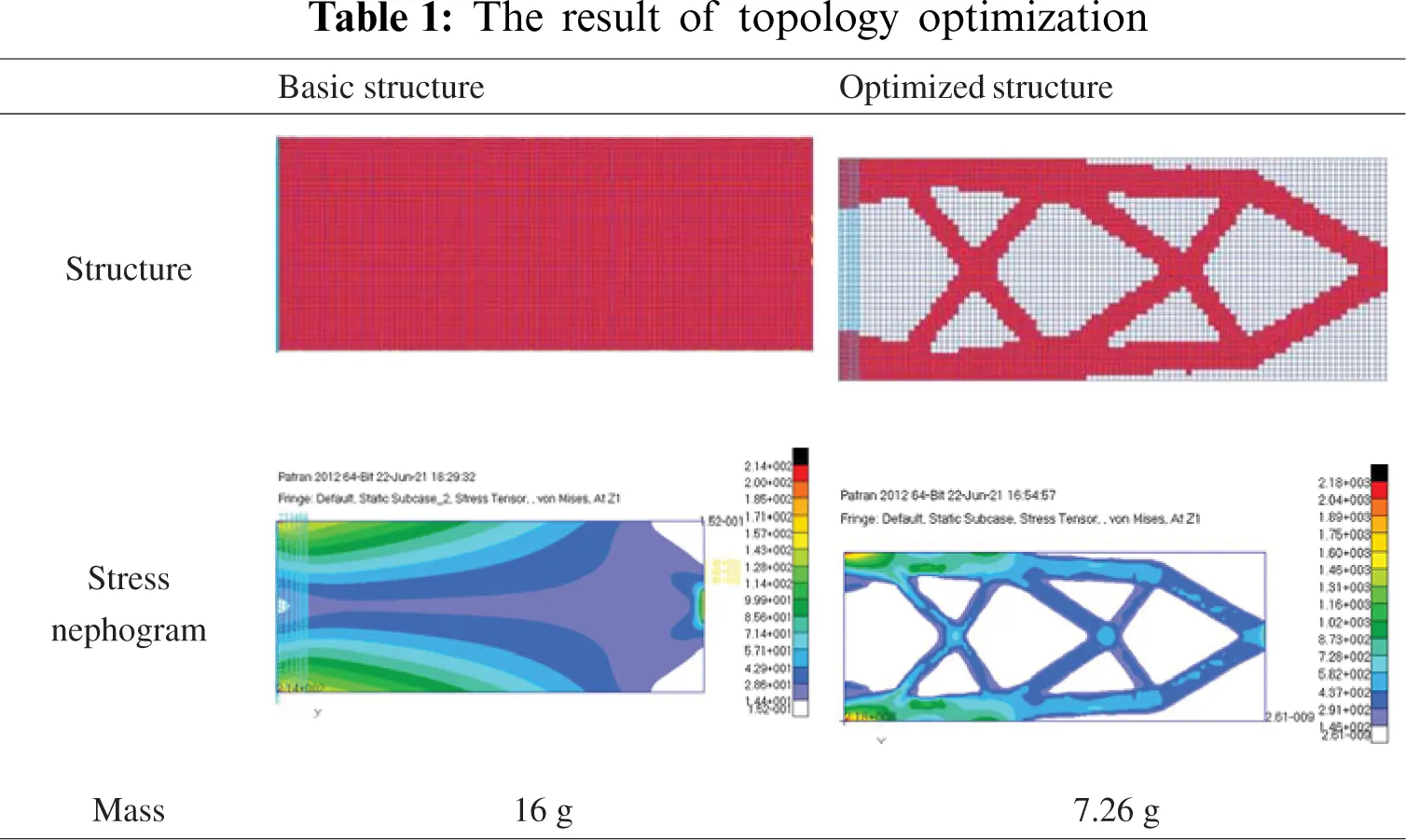Comparing the mass iteration history in Fig. 4B and Tab. 1, the mass of continuous optimized structure is less than the discrete optimized structure. This is because there are many intermediate topology variables, and the discretization of the intermediate variables is not very good. With the fatigue topology optimization, we obtain the conservative optimized structure.

In Tab. 2, the optimized structures obtained by different fatigue topology optimization methods for the same basic structure are compared. The optimized structures have similar configuration, which demonstrates the validity of ICM method. The efficiency of the ICM method is reflected in the number of iterations, that is 22 in ICM method, and more than 300 in SIMP method .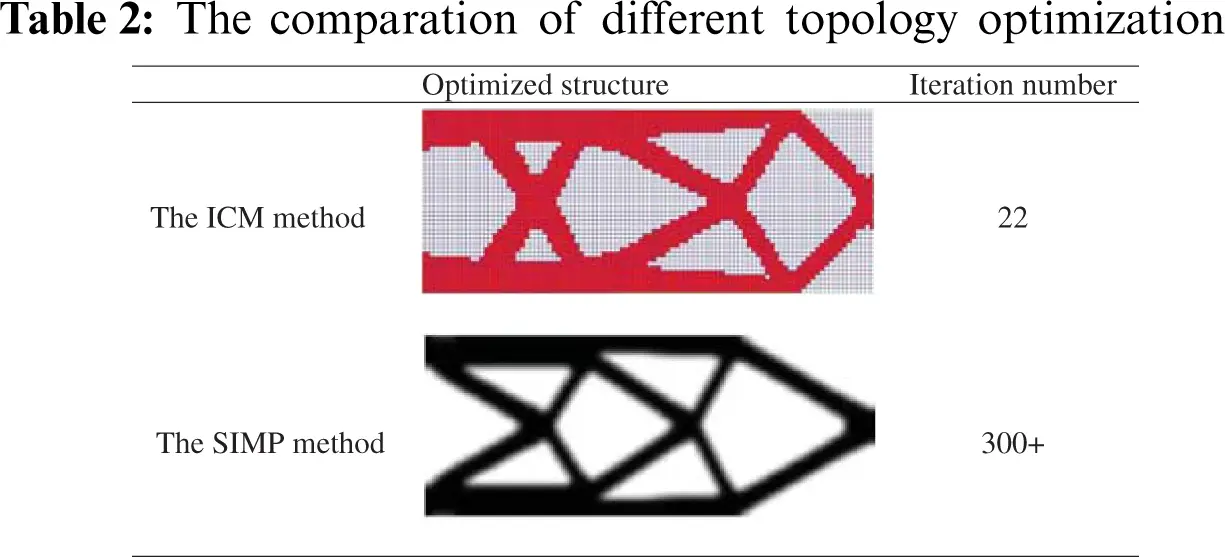6.2 Example 2

In Example 2, the basic structure is a simply supported beam with the size of 80 mm×20 mm×2 mm. The peak value of cyclic load is F = 3600 N. ρ = 1 kg/cm3. The total mass of the basic structure is 3200 g. The convergence precision ε = 0.01 in this example. The fatigue life of two units with the displacement constraints is infinite to reduce the effects of stress concentration.

From Fig. 5B, the mass iterative process gradually converges until the convergence precision is satisfied. To reveal the influence of different fatigue life constraints on the topological structure, 850, 900 and 950 are selected as the fatigue life constraints. Tab. 3 gives the fatigue life constraint, the structural configuration, mass and the optimized structure fatigue life in three cases.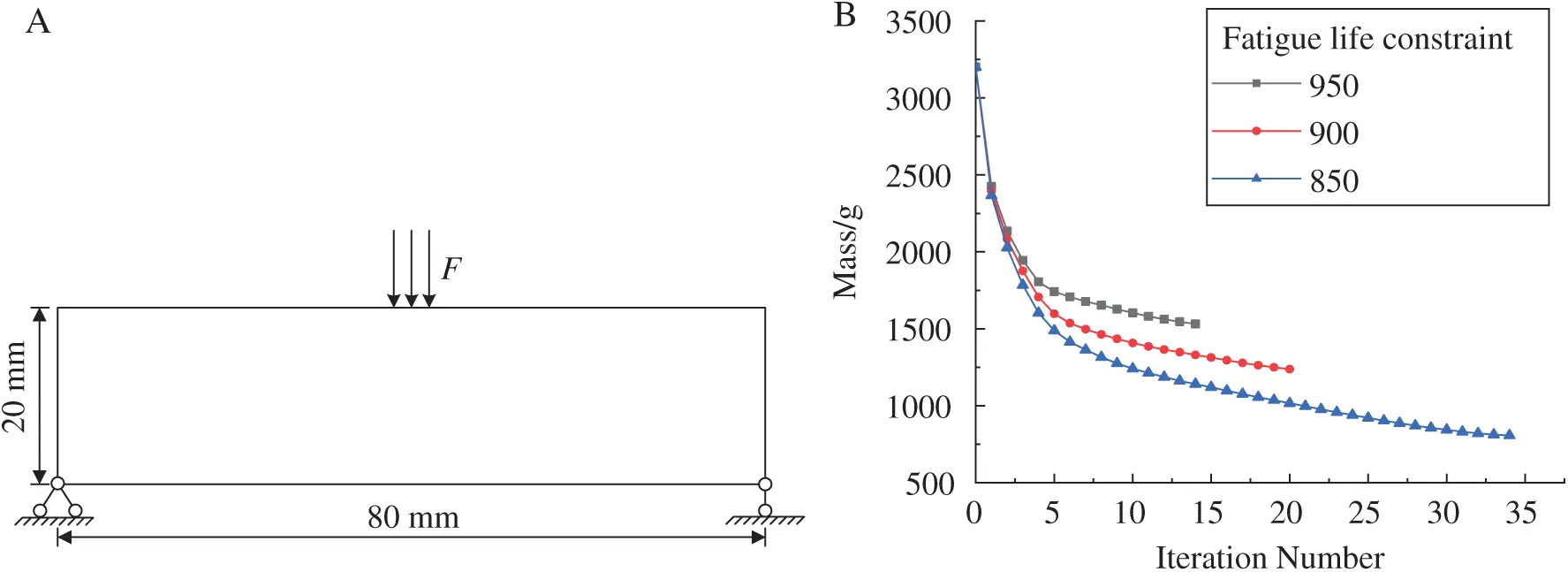Figure 5: (A) Basic structure. (B) Iterative curves of mass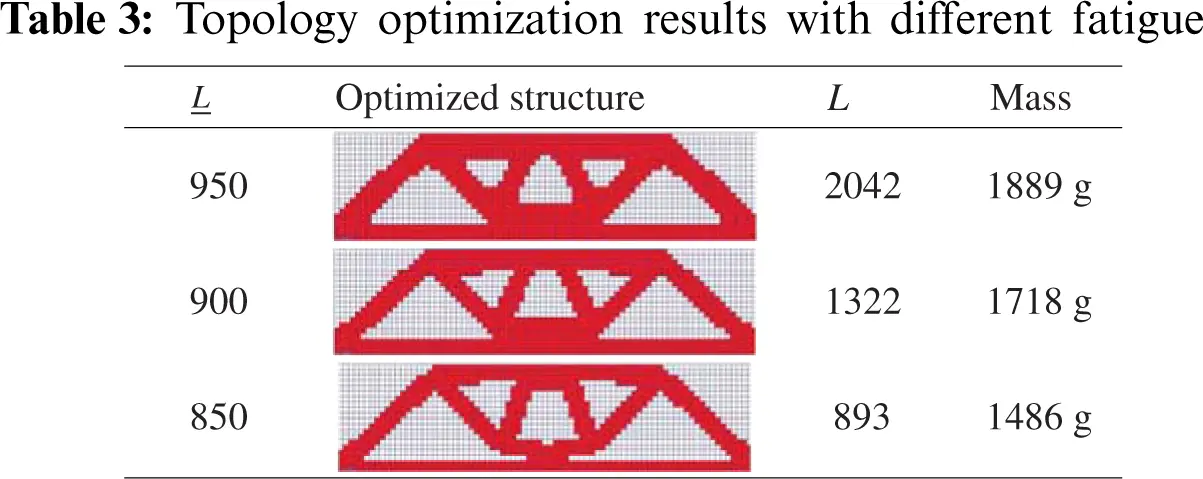From Tab. 3, the topology configurations are similar in all conditions, and the optimized structure fatigue life satisfies the constraint in all cases. When the fatigue life constraint is 950, the optimized structure fatigue life is 2042, the optimized structure mass is 1889 g, and the mass loss is 40.97%. When the fatigue life constraint is 900, the optimized structure fatigue life is 1322, the optimized structure mass is 1718 g, the mass loss is 46.31%. When the fatigue life constraint is 850, the optimized structure fatigue life is 893, the optimized structure mass is 1486 g and the mass loss is 53.56%. With the decrease of the fatigue life constraints, the fatigue life and the mass of optimized structure reduce gradually. From the detailed data, the fatigue life constraint decreases from 950 to 850. The reduce proportion of fatigue life constraint is 10.53%. At the same time, the structural mass decreases from 2042 g to 893 g. The reduce proportion of structural mass is 56.27%.

6.3 Example 3

The design domain is a beam structure of 80 mm×20 mm×2 mm as shown in Fig. 6A. ρ = 1 kg/cm3. The original structure mass is 3200 g. A cyclic load is applied on the middle part of the upper side, the left and right sides are fixed. The force is applied to three nodes to avoid the stress concentration. The fatigue life constraint is 150.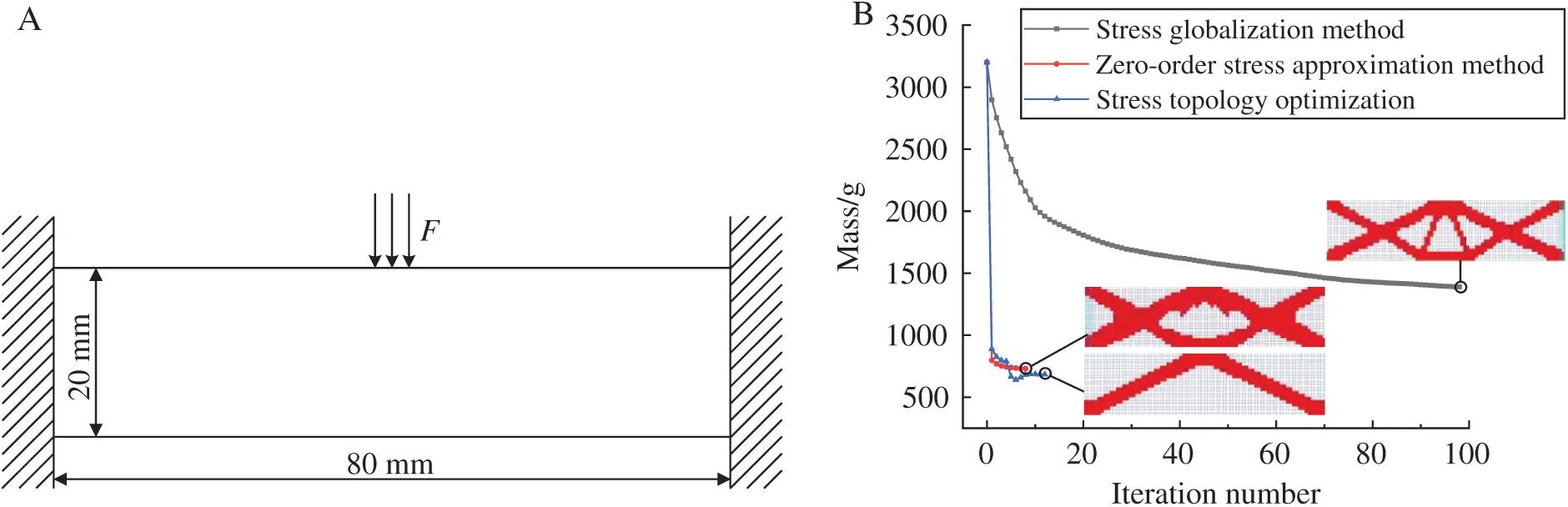Figure 6: (A) Basic structure. (B) Mass iteration curves of different topology optimizationsTo compare the differences between fatigue topology optimization and traditional stress optimization . The zero-order stress approximation  and distortion energy theory are introduced in fatigue topology optimization under the cyclic load with the peak value F = 5850 N, and the traditional stress optimization is carried out under the fixed static load F = 5850 N. Tab. 4 shows the comparison between fatigue topology optimization and stress topology optimization. Fig. 6B shows the mass iteration history curves of the two optimizations. The convergence precision and fatigue life constraints are satisfied in all three topology optimizations.

From Tab. 4, in the iteration Step 8, the optimized structure configuration with the distortion energy theory is the most complicated, followed by the optimized structure with the zero-order stress approximation, and the simple configuration is the optimized structure with the stress optimization. The same result can be obtained from the final optimized structures. We can observe that the simple configuration under stress optimization can be found in all conditions, which is the part of optimized structure in fatigue topology optimizations. Based on the configuration of stress topology optimization, the fatigue topology optimization configurations retain more materials and add some new force transmission paths. In other words, the optimized structures with fatigue life constraints are more conservative.

7  Conclusion

In this paper, the fatigue topology optimization is presented based on ICM method and fatigue analysis method. The lightweight topology optimization model is established, which uses fatigue life as constraint. The fatigue life constraints are transformed into distortion energy constraints with the S-N curve and the distortion energy theory. The effectiveness and validity of fatigue optimization method are verified by the comparation between the ICM method and the SIMP method. The numerical examples demonstrate the lightweight topology optimization design with the fatigue constraint can be achieved by the presented method.

In addition, the Miner rule and the S-N curve is carried out in this paper. In the future, we can discuss effect on the structural topological configuration according to other different fatigue failure criteria.

Funding Statement: This work was supported by the National Natural Science Foundation of China (11872080), and Beijing Natural Science Foundation (3192005).

Conflicts of Interest: The authors declare that they have no conflicts of interest to report regarding the present study.

## References

1. Wei, P., Wang, W. W., Yang, Y., & Wang, M. Y. (2020). Level set band method: A combination of density-based and level set methods for the topology optimization of continuums. Frontiers of Mechanical Engineering, 15(3), 390-405. [Google Scholar] [CrossRef]
2. Zhou, K. M. (2015). Topology optimization of prager structures based on truss-like material model. Structural and Multidisciplinary Optimization, 51, 1077-1081. [Google Scholar] [CrossRef]
3. Zhou, L., & Zhang, W. (2019). Topology optimization method with elimination of enclosed voids. Structural and Multidisciplinary Optimization, 60, 117-136. [Google Scholar] [CrossRef]
4. Lv, Y., & Liu, S. (2018). Topology optimization and heat dissipation performance analysis of a micro-channel heat sink. Meccanica, 53, 3693-3708. [Google Scholar] [CrossRef]
5. Zhu, J. H., Zhang, W. H., & Xia, L. (2016). Topology optimization in aircraft and aerospace structures design. Archives of Computational Methods in Engineering, 23, 595-622. [Google Scholar] [CrossRef]
6. Bendsøe, M. P., & Sigmund, O. (1999). Material interpolate-on schemes in topology optimization. Archive of Applied Mechanics, 69(9–10), 635-654. [Google Scholar] [CrossRef]
7. Bendsøe, M. P., & Kikuchi, N. (1988). Generating optimal topologies in structural design using a homogenization method. Computer Methods in Applied Mechanics and Engineering, 71(2), 197-224. [Google Scholar] [CrossRef]
8. Xie, Y. M., & Steven, G. P. (1993). A simple evolutionary procedure for structural optimization. Computers & Structures, 49(5), 885-896. [Google Scholar] [CrossRef]
9. Osher, S., & Sethian, J. (1988). Fronts propagating with curvature-dependent speed: Algorithms based on hamilton-jacobi formulations. Journal of Computational Physics, 79(1), 12-49. [Google Scholar] [CrossRef]
10. Yaghmaei, M., & Ali, G. (2019). A level set topology optimization method using a biharmonic equation based on plate theory. Structural and Multidisciplinary Optimization, 60, 2431-2459. [Google Scholar] [CrossRef]
11. Cheng, K. T., & Olhoff, N. (1981). An investigation concerning optimal design of solid elastic plates. International Journal of Solid and Structure, 17(3), 305-323. [Google Scholar] [CrossRef]
12. Guo, X., Zhang, W. Z., & Zhong, W. L. (2014). Doing topology optimization explicitly and geometrically—A new moving morphable components based framework. Journal of Applied Mechanics, 81(8), 081009. [Google Scholar] [CrossRef]
13. Guo, X., Zhang, W. S., & Zhang, J. (2016). Explicit structural topology optimization based on moving morphable components (MMC) with curved skeletons. Computer Methods in Applied Mechanics and Engineering, 310, 711-748. [Google Scholar] [CrossRef]
14. Zhang, W. S., & Li, D. D. (2019). Explicit topology optimization using IGA-based moving morphable void (MMV) approach. Computer Methods in Applied Mechanics and Engineering, 360, 112685. [Google Scholar] [CrossRef]
15. Akihiro, T., Nishiwaki, S., & Kitamura, M. (2010). Shape and topology optimization based on the phase field method and sensitivity analysis. Journal of Computational Physics, 229, 2697-2718. [Google Scholar] [CrossRef]
16. Jeong, S. H., Yoon, G. H., & Akihiro, T. (2014). Development of a novel phase-field method for local stress-based shape and topology optimization. Computers and Structures, 132, 84-98. [Google Scholar] [CrossRef]
17. Sui, Y. K., Peng, X. R. (2017). Modeling, solving and application for topology optimization of continuum structures: ICM method based on step function. Beijing, Tsinghua University Press.
18. Mrzyglod, M., & Zielinski, A. P. (2007). Multiaxial high-cycle fatigue constraints in structural optimization. International Journal of Fatigue, 29(9–11), 1920-1926. [Google Scholar] [CrossRef]
19. Desmorat, B., & Desmorat, R. (2007). Topology optimization in damage governed low cycle fatigue. Comptes Rendus Mecanique, 336(5), 448-453. [Google Scholar] [CrossRef]
20. Suresh, S., & Lindström, B. (2020). Topology optimization using a continuous-time high-cycle fatigue model. Structural and Multidisciplinary Optimization, 61(3), 1011-1025. [Google Scholar] [CrossRef]
21. Suresh, S., & Lindström, B. (2021). Topology optimization for transversely isotropic materials with high-cycle fatigue as a constraint. Structural and Multidisciplinary Optimization, 63, 161-172. [Google Scholar] [CrossRef]
22. Holmberg, E., Torstenfelt, B., & Klarbring, A. (2014). Fatigue constrained topology optimization. Structural and Multidisciplinary Optimization, 50(2), 207-219. [Google Scholar] [CrossRef]
23. Oest, J., & Lund, E. (2017). Topology optimization with finite-life fatigue constraints. Structural and Multidisciplinary Optimization, 56(5), 1045-1059. [Google Scholar] [CrossRef]
24. Lee, J. W., Yoon, G. H., & Jeong, S. H. (2015). Topology optimization considering fatigue life in the frequency domain. Computers and Mathematics with Applications, 70(8), 1852-1877. [Google Scholar] [CrossRef]
25. Chen, Z., Long, K., Wen, P., & Nouman, S. (2020). Fatigue-resistance topology optimization of continuum structure by penalizing the cumulative fatigue damage. Advances in Engineering Software, 150, 102924. [Google Scholar] [CrossRef]
26. Nabaki, K., Shen, J., & Huang, X. D. (2019). Evolutionary topology optimization of continuum structures considering fatigue failure. Material and Design, 166, 107586. [Google Scholar] [CrossRef]
27. Collect, M., Bruggi, M., & Duysinx, P. (2017). Topology optimization for minimum weight with compliance and simplified nominal stress constraints for fatigue resistance. Structural and Multidisciplinary Optimization, 55, 839-855. [Google Scholar] [CrossRef]
28. París, J., Navarrina, F., Colominas, I., & Casteleiro, M. (2009). Topology optimization of continuum structures with local and global stress constraints. Structural and Multidisciplinary Optimization, 39(4), 419-437. [Google Scholar] [CrossRef]
29. Ye, H. L., Wang, W. W., & Chen, N. (2016). Plate/shell topological optimization subjected to linear buckling constraints by adopting composite exponential filtering function. Acta Mechanica Sinica, 32(4), 649-658. [Google Scholar] [CrossRef]
30. Du, J. Z., Guo, Y. H., Chen, Z. M., & Sui, Y. K. (2019). Topology optimization of continuum structures considering damage based on independent continuous mapping method. Acta Mechanica Sinica, 35(2), 433-444. [Google Scholar] [CrossRef]This work is licensed under a Creative Commons Attribution 4.0 International License, which permits unrestricted use, distribution, and reproduction in any medium, provided the original work is properly cited.